#### How to Use the Trapezoidal Rule in Excel

This is what it is and how to use the trapezoidal rule in Excel.

## What Is The Trapezoidal Rule?

The trapezoidal rule is a numerical method for approximating the definite integral of a function. We use the trapezoidal rule to estimate the integral of a function; that is to estimate the value of intervals of one or more variables.

The rule works by evaluating the area under a curve after first dividing the total area into smaller trapezoids. From there, we can calculate the area.

## Why Use the Trapezoidal Rule in Excel?

The trapezoidal rule is a versatile tool that can be used to approximate the definite integral of a wide variety of functions. It is also a relatively simple method to implement in Excel, making it a good choice for beginners.

## How to calculate the Trapezoidal Rule?

The steps below will take you through the process to create a table to chart a curve. A quick reference guide is available at the end of this document.

Open the spreadsheet How To Use The Trapezoidal Rule In Excel – Sample Data, go to Sheet 1.

Create a table containing the variables and their range. We will use the values 0 – 6 as our limits of integration.

Determine for each interval how many subintervals you want. We will use 2 subintervals per interval or 1/2 =.5. The more slices used increases the accuracy of the result.

Using the subintervals identified enter the range of values in the column with the heading ‘x‘. Example list the values 1 through x, x being the upper value of the range you want to analyze.

Make the following entries: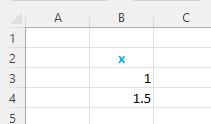• B2x, this is the column heading
• B31
• B45

Select both B3 and B4, left click the fill handle then drag the handle down to B13. This covers the range we identified.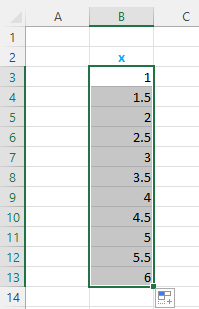Label the next column. In C2, type the column heading f(x)=1/x; the formula for this column.

Divide 1 by each of the intervals. In C3 type =1/B3, press enter.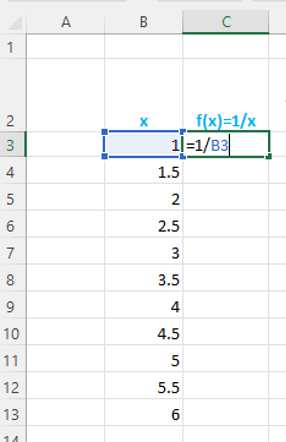Use the fill handle to copy this formula to the cells of this column.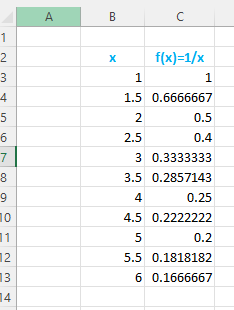Label the next column. In D2 type the heading Area for each interval.

Under the heading skip the first cell, in the next cell (D4).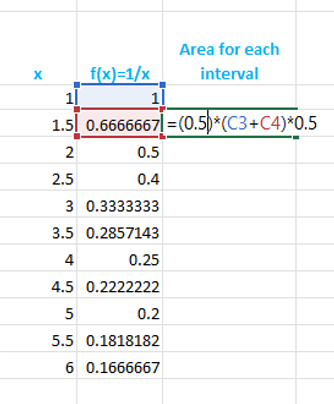Type this formula =(0.5)*(C3+C4)*0.5.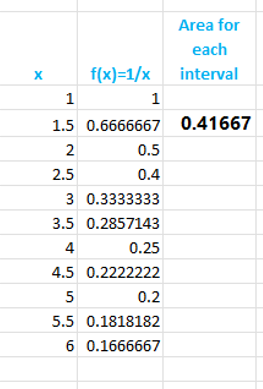Use the fill handle to copy to the rest of this column.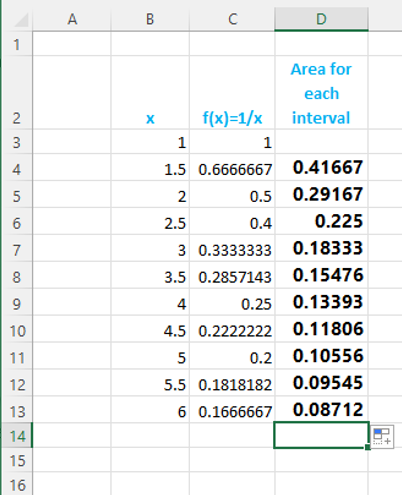Total the values in the Area for each interval.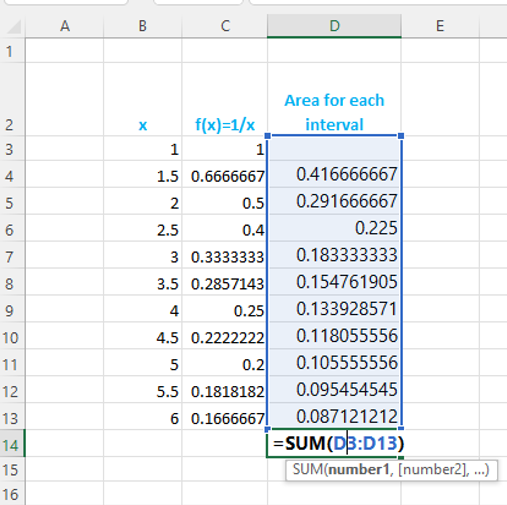In cell D14 type =SUM(D3:D13), press enter.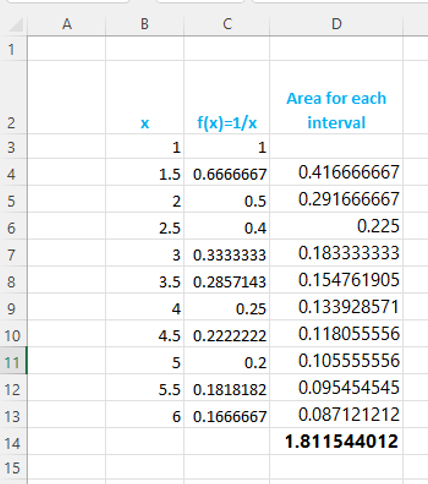## Comparison with Other Numerical Methods

The trapezoidal rule is a simple and accurate way to approximate the definite integral of a function. However, there are other numerical methods that can be used as well.

One alternative method is the Simpson’s rule. The Simpson’s rule is more accurate than the trapezoidal rule, but it is also more complex to implement.

Another alternative method is the Midpoint rule. The Midpoint rule is less accurate than the trapezoidal rule, but it is also simpler to implement.

The following table compares the three methods:

Method Accuracy Complexity
Trapezoidal rule Less accurate Simple
Simpson’s rule More accurate Complex
Midpoint rule Least accurate Simplest

The best method to use depends on the specific application. If accuracy is important, then the Simpson’s rule is the best choice. If simplicity is important, then the trapezoidal rule or the Midpoint rule may be better choices.

## Quick reference guide

1. We will use specific cell addresses in this guide to make the steps easier to follow.